Get instant live expert help with Excel or Google Sheets“My Excelchat expert helped me in less than 20 minutes, saving me what would have been 5 hours of work!”

#### Post your problem and you’ll get Expert help in seconds.

Your message must be at least 40 characters
Our professional Expert are available now. Your privacy is guaranteed.

# How to Use the DEVSQ Function

We can use the DEVSQ function to calculate the sum of squares of deviations from the mean value of a data range. The steps below will walk through the process.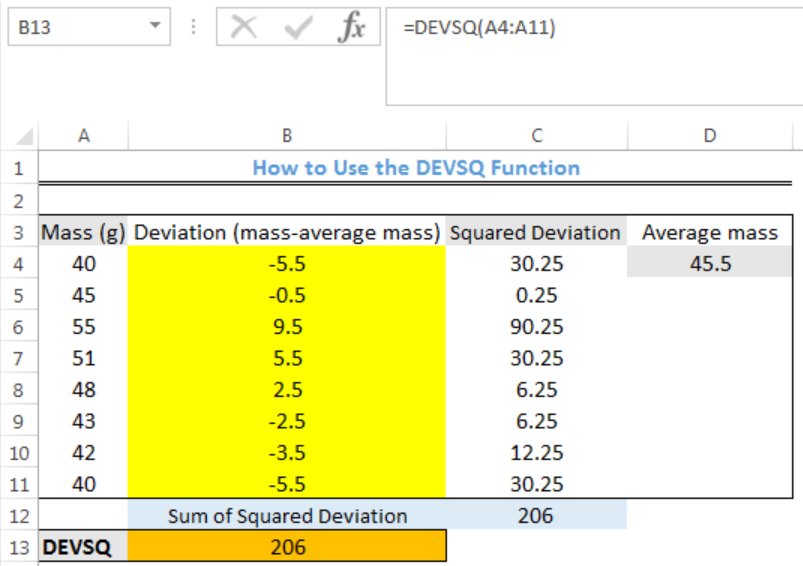Figure 1: How to Use the DEVSQ Function

## Syntax

`=DEVSQ([number1], [number2], [number2] ...)`

## Formula

`=DEVSQ(A4:A11)`

## Setting up the Data

• We will set up the data by inputting the MASS into Column A
• We will put the deviation of the values in Column B
• Column C will contain the squared deviation of the values
• Column D contains the average mass
• Cell B13 is where our result with the DEVSQ function will  be returned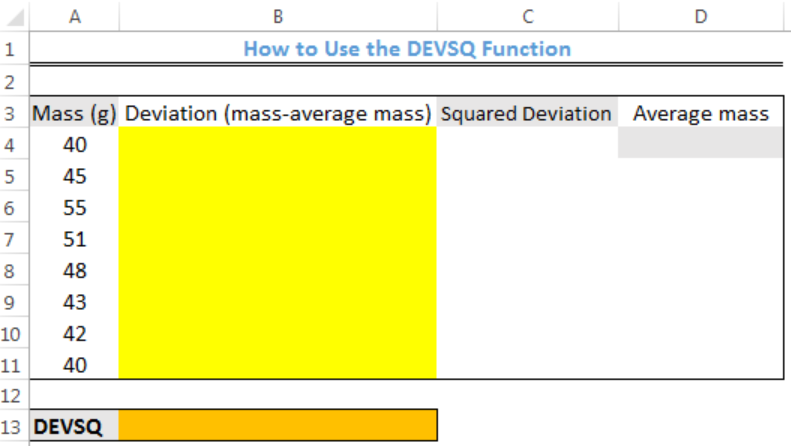Figure 2: Setting up the Data

## Using the DEVSQ function

• We will click on Cell B13
• We will insert the formula below into the cell
`=DEVSQ(A4:A11)`
• We will press the enter key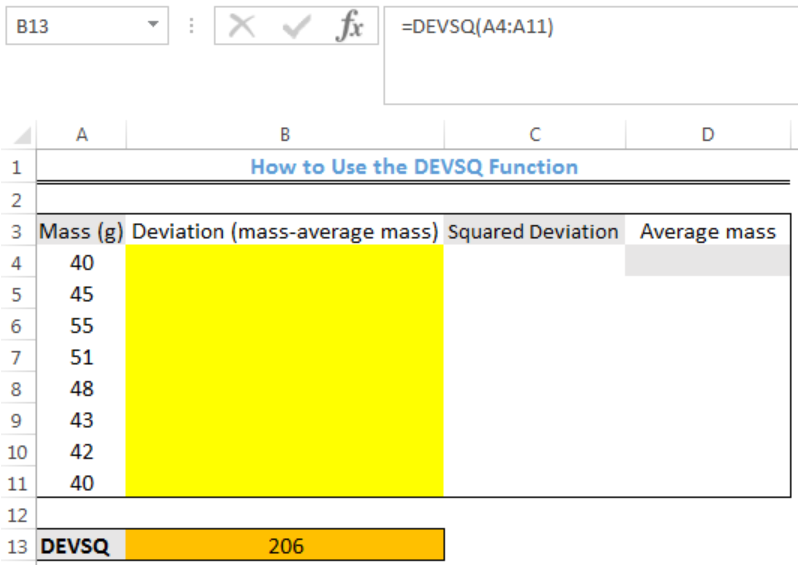Figure 3: Result with the DEVSQ Function

## Alternative Long Method

### Calculating the Average Mass

• We will click on Cell D4
• We will insert the formula below into the cell
`=AVERAGE(A4:A11)`
• We will press the enter key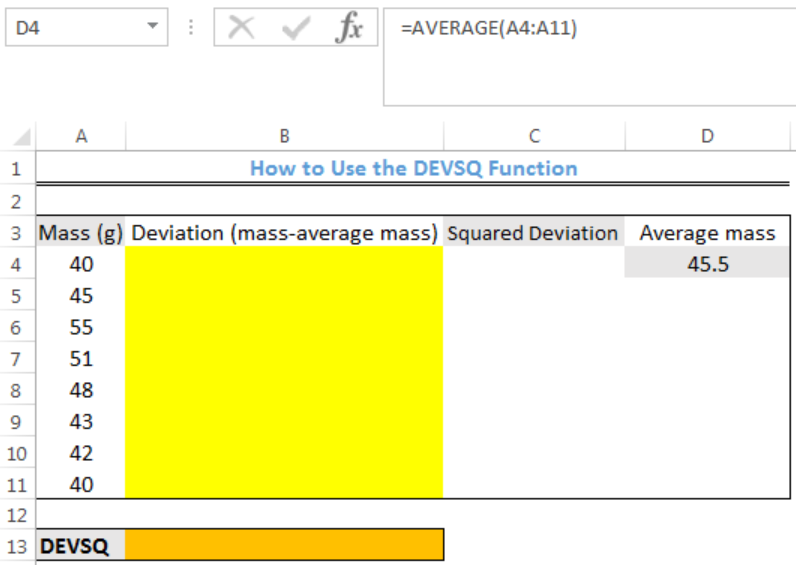Figure 4: Average Mass of the Data

### Calculating the Deviation

• We will click on Cell B4
• We will insert the formula below into the cell
`=A4-\$D\$4`
• We will press the enter key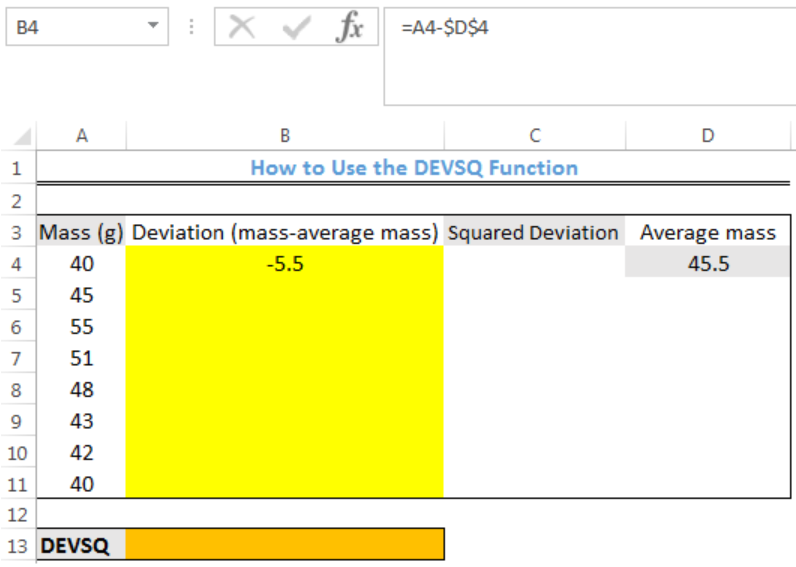Figure 5: Deviation Value for Data in Cell A4

• We will click on Cell B4 again
• We will double-click on the fill handle (the small plus sign at the bottom right of Cell B4) and drag down to copy the formula into the other cells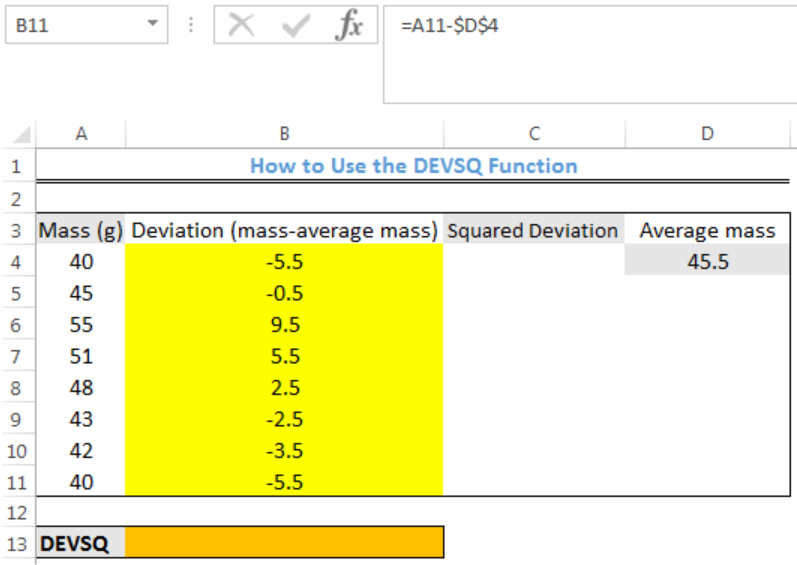Figure 6: Deviation Values

### Calculating the Squared Deviation

• We will click on Cell C4
• We will insert the formula below into the cell
`=B4^2`
• We will press the enter key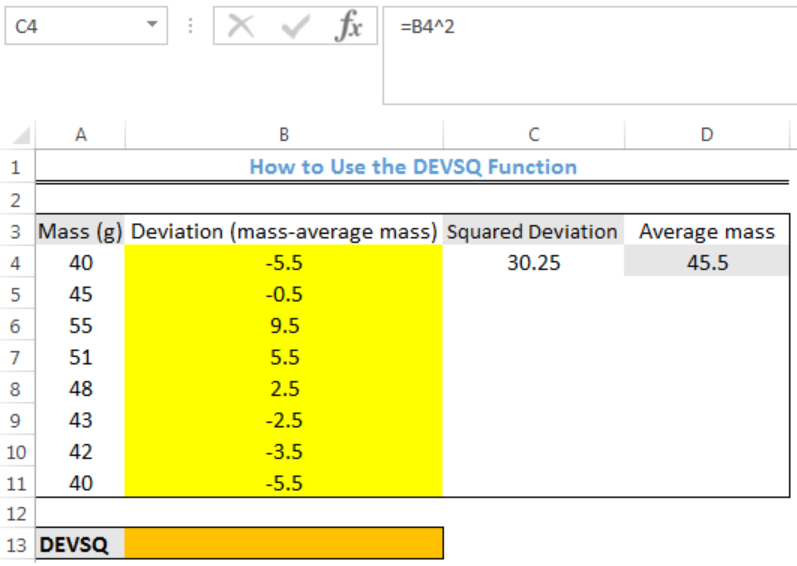Figure 7: Squared Deviation Value for Data in Cell B4

• We will click on Cell C4 again
• We will double-click on the fill handle (the small plus sign at the bottom right of Cell C4) and drag down to copy the formula into the other cells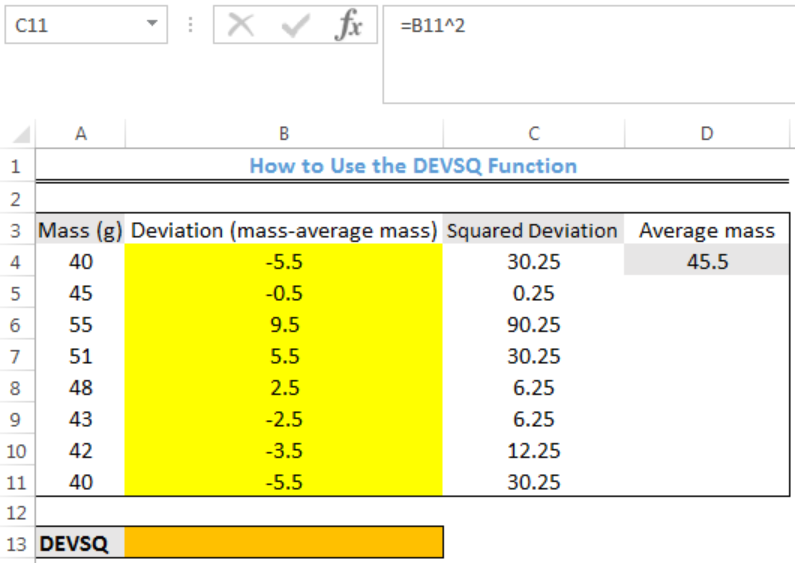Figure 8: Squared Deviation Values

• We will sum the squared deviation by inserting the formula below into Cell C12
`=SUM(C4:C11)`
• We will press the enter key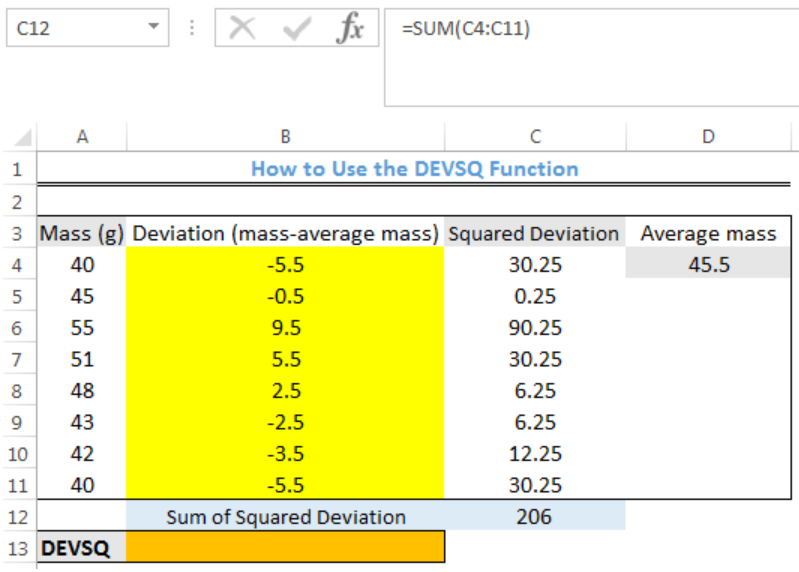Figure 9: Sum of Squared Deviation Values

We can see that the result from the alternative method which is longer is the same with the first method.

## Instant Connection to an Expert through our Excelchat Service

Most of the time, the problem you will need to solve will be more complex than a simple application of a formula or function. If you want to save hours of research and frustration, try our live Excelchat service! Our Excel Experts are available 24/7 to answer any Excel question you may have. We guarantee a connection within 30 seconds and a customized solution within 20 minutes.